Discrete Random Variables

# 21 Hypergeometric Distribution

The simplest probability density function is the hypergeometric. This is the most basic one because it is created by combining our knowledge of probabilities from Venn diagrams, the addition and multiplication rules, and the combinatorial counting formula.

To find the number of ways to get 2 aces from the four in the deck we computed: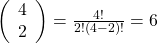And if we did not care what else we had in our hand for the other three cards we would compute: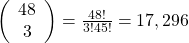Putting this together, we can compute the probability of getting exactly two aces in a 5 card poker hand as: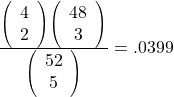This solution is really just the probability distribution known as the Hypergeometric. The generalized formula is: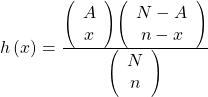where x = the number we are interested in coming from the group with A objects.

h(x) is the probability of x successes, in n attempts, when A successes (aces in this case) are in a population that contains N elements. The hypergeometric distribution is an example of a discrete probability distribution because there is no possibility of partial success, that is, there can be no poker hands with 2 1/2 aces. Said another way, a discrete random variable has to be a whole, or counting, number only. This probability distribution works in cases where the probability of a success changes with each draw. Another way of saying this is that the events are NOT independent. In using a deck of cards, we are sampling WITHOUT replacement. If we put each card back after it was drawn then the hypergeometric distribution be an inappropriate Pdf.

For the hypergeometric to work,

1. the population must be dividable into two and only two independent subsets (aces and non-aces in our example). The random variable X = the number of items from the group of interest.
2. the experiment must have changing probabilities of success with each experiment (the fact that cards are not replaced after the draw in our example makes this true in this case). Another way to say this is that you sample without replacement and therefore each pick is not independent.
3. the random variable must be discrete, rather than continuous.

A candy dish contains 30 jelly beans and 20 gumdrops. Ten candies are picked at random. What is the probability that 5 of the 10 are gumdrops? The two groups are jelly beans and gumdrops. Since the probability question asks for the probability of picking gumdrops, the group of interest (first group A in the formula) is gumdrops. The size of the group of interest (first group) is 30. The size of the second group is 20. The size of the sample is 10 (jelly beans or gumdrops). Let X = the number of gumdrops in the sample of 10. X takes on the values x = 0, 1, 2, …, 10. a. What is the probability statement written mathematically? b. What is the hypergeometric probability density function written out to solve this problem? c. What is the answer to the question “What is the probability of drawing 5 gumdrops in 10 picks from the dish?”

a.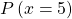b.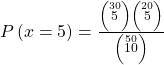c.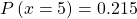Try It

A bag contains letter tiles. Forty-four of the tiles are vowels, and 56 are consonants. Seven tiles are picked at random. You want to know the probability that four of the seven tiles are vowels. What is the group of interest, the size of the group of interest, and the size of the sample?

The group of interest is the vowel letter tiles. The size of the group of interest is 44. The size of the sample is seven.

### Chapter Review

The combinatorial formula can provide the number of unique subsets of size x that can be created from n unique objects to help us calculate probabilities. The combinatorial formula is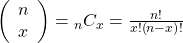A hypergeometric experiment is a statistical experiment with the following properties:

1. You take samples from two groups.
2. You are concerned with a group of interest, called the first group.
3. You sample without replacement from the combined groups.
4. Each pick is not independent, since sampling is without replacement.

The outcomes of a hypergeometric experiment fit a hypergeometric probability distribution. The random variable X = the number of items from the group of interest..

### Formula ReviewUse the following information to answer the next five exercises: Suppose that a group of statistics students is divided into two groups: business majors and non-business majors. There are 16 business majors in the group and seven non-business majors in the group. A random sample of nine students is taken. We are interested in the number of business majors in the sample.

In words, define the random variable X.

X = the number of business majors in the sample.

What values does X take on?

2, 3, 4, 5, 6, 7, 8, 9

### HOMEWORK

A group of Martial Arts students is planning on participating in an upcoming demonstration. Six are students of Tae Kwon Do; seven are students of Shotokan Karate. Suppose that eight students are randomly picked to be in the first demonstration. We are interested in the number of Shotokan Karate students in that first demonstration.

1. In words, define the random variable X.
2. List the values that X may take on.
3. How many Shotokan Karate students do we expect to be in that first demonstration?

<!– <solution id=”fs-idp3312224″> X = the number of Shotokan Karate students in the first demonstration 0, 1, 2, 3, 4, 5, 6, 7 4.31 –>

In one of its Spring catalogs, L.L. Bean® advertised footwear on 29 of its 192 catalog pages. Suppose we randomly survey 20 pages. We are interested in the number of pages that advertise footwear. Each page may be picked at most once.

1. In words, define the random variable X.
2. List the values that X may take on.
3. How many pages do you expect to advertise footwear on them?
4. Calculate the standard deviation.
1. X = the number of pages that advertise footwear
2. 0, 1, 2, 3, …, 20
3. 3.03
4. 1.5197

Suppose that a technology task force is being formed to study technology awareness among instructors. Assume that ten people will be randomly chosen to be on the committee from a group of 28 volunteers, 20 who are technically proficient and eight who are not. We are interested in the number on the committee who are not technically proficient.

1. In words, define the random variable X.
2. List the values that X may take on.
3. How many instructors do you expect on the committee who are not technically proficient?
4. Find the probability that at least five on the committee are not technically proficient.
5. Find the probability that at most three on the committee are not technically proficient.

<!– <solution id=”eip-idm90541104″> X = the number of people on the committee who are not technically proficient 0, 1, 2, 3, 4, 5, 6, 7, 8, 9, 10 2.8571 0.1269 0.6873 –>

Suppose that nine Massachusetts athletes are scheduled to appear at a charity benefit. The nine are randomly chosen from eight volunteers from the Boston Celtics and four volunteers from the New England Patriots. We are interested in the number of Patriots picked.

1. In words, define the random variable X.
2. List the values that X may take on.
3. Are you choosing the nine athletes with or without replacement?
1. X = the number of Patriots picked
2. 0, 1, 2, 3, 4
3. Without replacement

A bridge hand is defined as 13 cards selected at random and without replacement from a deck of 52 cards. In a standard deck of cards, there are 13 cards from each suit: hearts, spades, clubs, and diamonds. What is the probability of being dealt a hand that does not contain a heart?

1. What is the group of interest?
2. How many are in the group of interest?
3. How many are in the other group?
4. Let X = _________. What values does X take on?
5. The probability question is P(_______).
6. Find the probability in question.
7. Find the (i) mean and (ii) standard deviation of X.

<!– <solution id=”fs-idp25943152″> Cards that are not hearts 52 − 13 = 39 Other group = hearts; there are 13 hearts in a deck. X = the number of cards that are not hearts; x = 0, 1, 2, 3, … , 13 P(x = 13) ( 39 52 )( 38 51 )( 37 50 )( 36 49 )( 35 48 )( 34 47 )( 33 46 )( 32 45 )( 31 44 )( 30 43 )( 29 42 )( 28 41 )( 27 40 ) ≈ 0.0128 Mean = μ = nr r + b = (13)(39) 39 + 13 = 9.75 Standard Deviation = σ = rbn(r + b−n) (r + b) 2 (r + b−1) = 39(13)13(39 + 13–13) (39 + 13) 2 (39 + 13–1) ≈ 1.3653 Alternate Solution Set Up Cards that are hearts 13 Other group = non-hearts; b = 39 X = the number of cards that are hearts; x = 0, 1, 2, 3, … , 13 P(x = 0)

### Key Terms

Hypergeometric Experiment
a statistical experiment with the following properties:

1. You take samples from two groups.
2. You are concerned with a group of interest, called the first group.
3. You sample without replacement from the combined groups.
4. Each pick is not independent, since sampling is without replacement.
Hypergeometric Probability
a discrete random variable (RV) that is characterized by:

1. A fixed number of trials.
2. The probability of success is not the same from trial to trial.

We sample from two groups of items when we are interested in only one group. X is defined as the number of successes out of the total number of items chosen.